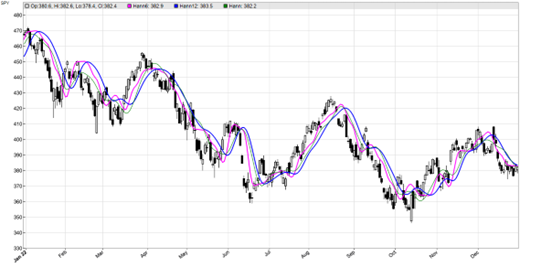# UndersamplingAll the popular ‘smoothing’ indicators, like SMA or lowpass filters, exchange more lag for more smoothing. In TASC 4/2023, John Ehlers suggested the undersampling of price curves for achieving a better compromise between smoothness and lag. We will check that by applying a Hann filter to the original price curve and to a 5-fold undersampled curve.

The C code of the used Hann filter, straight from Ehler’s article:

```var Hann(vars Data,int Length)
{
var Filt = 0, Coeff = 0;
int i; for(i=1; i<=Length; i++) {
Filt += (1-cos(2*PI*i/(Length+1)))*Data[i-1];
Coeff += 1-cos(2*PI*i/(Length+1));
}
return Filt/fix0(Coeff);
}
```

The fix0 function in the denominator is a convenience function for fixing division by zero issues.

We will now apply this Hann filter to the undersampled SPY price curve. Undersampling means: Take only every n-th price, and throw the rest away. We use the modulo operator to detect every 5th bar:

```void run()
{
StartDate = 20220101;
EndDate = 20221231;
BarPeriod = 1440;
set(PLOTNOW);
asset("SPY");
vars Samples = series();
if(Init || Bar%5 == 0) // sample only every 5th bar
Samples = priceC(0);
else
Samples = Samples;
plot("Hann6",Hann(Samples,6),LINE,MAGENTA);
plot("Hann12",Hann(Samples,12),LINE,BLUE);
plot("Hann",Hann(seriesC(),12),0,DARKGREEN);
}
```

The resulting chart:The blue line is the Hann filter of the undersampled curve with period 12, the magenta line with period 6. For comparison I’ve added the Hann filter output from the original curve (thin green line). We can see that the green line has less lag, but is also less smooth. Will using undersampled data improve a trading system? Well… I guess it depends on the system.

The Hann indicator and undersampling test script can be downloaded from the 2022 script repository.

## 4 thoughts on “Undersampling”

1.Arnis Lapsa says:

Failed to find a positive feedback with this one. I like the concept though.

2.Grant ahead says:

Thanks for sharing. How could I get the password for uncomprossing the 3rd bookscript of the black book?

3.jcl says:

Look in the file list of the zip file. There is a hint.

This site uses Akismet to reduce spam. Learn how your comment data is processed.